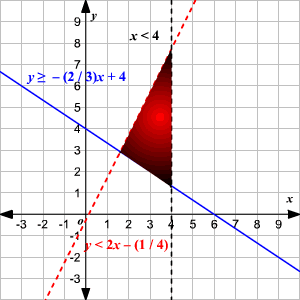# Graphing Systems of Linear Inequalities

To graph a linear inequality in two variables (say, $x$ and $y$ ), first get $y$ alone on one side. Then consider the related equation obtained by changing the inequality sign to an equality sign. The graph of this equation is a line.

If the inequality is strict ( $<$ or $>$ ), graph a dashed line. If the inequality is not strict ( $\le$ or $\ge$ ), graph a solid line.

Finally, pick one point that is not on either line ( $\left(0,0\right)$ is usually the easiest) and decide whether these coordinates satisfy the inequality or not. If they do, shade the half-plane containing that point. If they don't, shade the other half-plane.

Graph each of the inequalities in the system in a similar way. The solution of the system of inequalities is the intersection region of all the solutions in the system.

Example 1:

Solve the system of inequalities by graphing:

$\begin{array}{l}y\le x-2\\ y>-3x+5\end{array}$

First, graph the inequality $y\le x-2$ . The related equation is $y=x-2$ .

Since the inequality is $\le$ , not a strict one, the border line is solid.

Graph the straight line.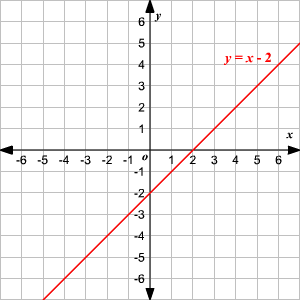Consider a point that is not on the line - say, $\left(0,0\right)$ - and substitute in the inequality $y\le x-2$ .

$\begin{array}{l}0\le 0-2\\ 0\le -2\end{array}$

This is false. So, the solution does not contain the point $\left(0,0\right)$ . Shade the lower half of the line.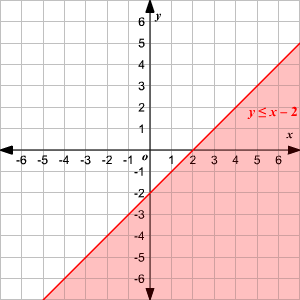Similarly, draw a dashed line for the related equation of the second inequality $y>-3x+5$ which has a strict inequality. The point $\left(0,0\right)$ does not satisfy the inequality, so shade the half that does not contain the point $\left(0,0\right)$ .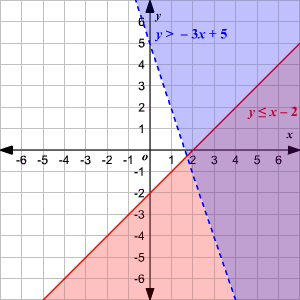The solution of the system of inequalities is the intersection region of the solutions of the two inequalities.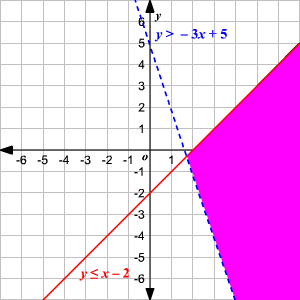Example 2:

Solve the system of inequalities by graphing:

$\begin{array}{l}2x+3y\ge 12\\ 8x-4y>1\\ x<4\end{array}$

Rewrite the first two inequalities with $y$ alone on one side.

$\begin{array}{cccc}\begin{array}{l}3y\ge -2x+12\\ y\ge -\frac{2}{3}x+4\end{array}& & & \begin{array}{l}-4y>-8x+1\\ y<2x-\frac{1}{4}\end{array}\end{array}$

Now, graph the inequality $y\ge -\frac{2}{3}x+4$ . The related equation is $y=-\frac{2}{3}x+4$ .

Since the inequality is $\ge$ , not a strict one, the border line is solid.

Graph the straight line.

Consider a point that is not on the line - say, $\left(0,0\right)$ - and substitute in the inequality.

$\begin{array}{l}0\ge -\frac{2}{3}\left(0\right)+4\\ 0\ge 4\end{array}$

This is false. So, the solution does not contain the point $\left(0,0\right)$ . Shade upper half of the line.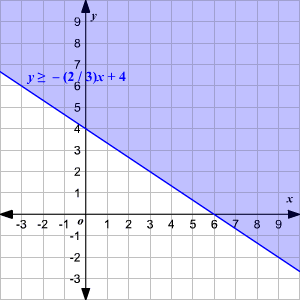Similarly, draw a dashed line of related equation of the second inequality $y<2x-\frac{1}{4}$ which has a strict inequality. The point $\left(0,0\right)$ does not satisfy the inequality, so shade the half that does not contain the point $\left(0,0\right)$ .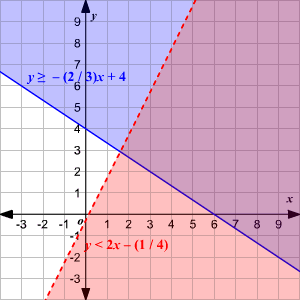Draw a dashed vertical line $x=4$ which is the related equation of the third inequality.

Here point $\left(0,0\right)$ satisfies the inequality, so shade the half that contains the point.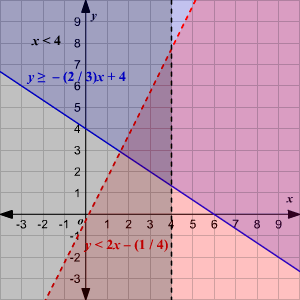The solution of the system of inequalities is the intersection region of the solutions of the three inequalities.